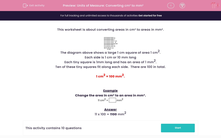# Units of Measure: Converting cm² to mm²

In this worksheet, students convert areas given in cm² to mm².Key stage:  KS 2

Curriculum topic:   Maths and Numerical Reasoning

Curriculum subtopic:   Metric & Imperial

Difficulty level:#### Worksheet Overview

This worksheet is about converting areas in cm² to areas in mm².The diagram above shows a large 1 cm square of area 1 cm2.
Each side is 1 cm or 10 mm long

Each tiny square is 1mm long and has an area of 1 mm2.

Ten of these tiny squares fit along each side.  There are 100 in total.

1 cm2 = 100 mm2.

Example

Change the area in cm² to an area in mm².

11 cm2
mm2